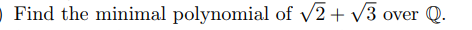the ninimal polynomial o 1V3 ovr

Question

Abstract Algebrahelp_outlineImage Transcriptionclosethe ninimal polynomial o 1V3 ovr fullscreen
Step 1

To find the smallest degree polynomial f(x) with coefficients in Q, such that f(√2+√3)=0.

Step 2

The important point is that the coefficients should all be in Q. For example, x-(√2+√3) is not allowed, as its coefficients are not rational numbers.

Step 3

We proceed to find this minimal polynomial by repeated squaring &...

Want to see the full answer?

See Solution

Want to see this answer and more?

Our solutions are written by experts, many with advanced degrees, and available 24/7

See Solution
Tagged in

Math